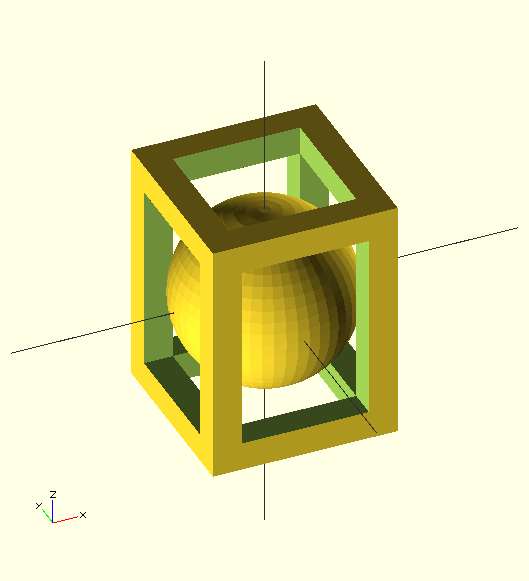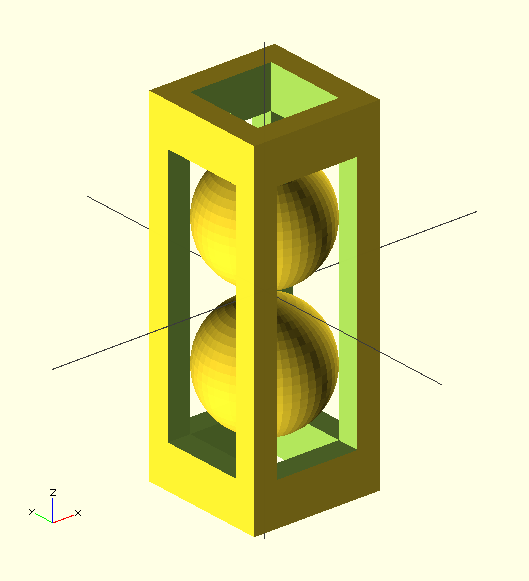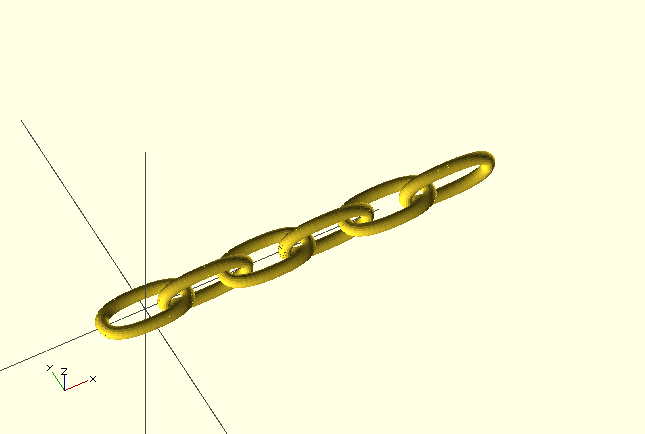Search

16 Mar 2013

Sailors over the ages have 'whittled' or carved wood with a pen-knife and I plan to take that up this summer. I think I will find it a useful distraction from the mild anxiety I suffer at sea. Whittling is the opposite of 3-D printing, progresssively removing material instead of adding it. There are some truely remarkable examples of complex interlocking objects carved from a single piece of softwood.

I thought it might be fun to use openSCAD to design artifacts for whittling. It is possible to create objects which are not easily printable in an extruder. so these designs are not intended for printing, but views of the artifact will be useful for blocking out the design and getting the proportions correct.

A traditional design is the ball in a cage.```module cage(size, width) {
assign(offset = 2 * width)
difference () {
cube(size, center=true);
cube([size - offset, size - offset, size + offset ], center=true);
cube([size - offset, size + offset,size - offset ], center=true);
cube([size + offset, size - offset,size - offset ], center=true);
}
};

module one_ball_cage (size, width) {
cage(size, width);
assign ( radius =( size - width *2) * cos(45) )
{
}
}

module two_ball_cage (size, width) {
cage(size, width);
assign ( radius =( size - width *2) * cos(45) )
assign ( gap = (size - (width * 2)) / 4 )
{
}
}

EPS=0.02;
\$fn=50;
*one_ball_cage([45,45,60],[7,7,7]);
two_ball_cage([45,45,120],[8,8,15]);

```

Another classic design is a chain..

The work on supercircles comes in handy here to create each link. The outline of the link is a superellipse with parameters adjusted to create a good-looking link. The hull() transform is used to create the segments which need to be inset to create a closed surface

```function normal (p1,p2) = [ p1 - p2, p2 - p1 ] ;
function length(p)  = sqrt(pow(p,2) + pow(p,2) );
function unit(p) = p / length(p);
function angle(p) = atan(p/p);

function superellipse (R, p, e, theta) =
R * [ e*    pow(abs(sin(theta)),2/p) * sign(sin(theta)),
pow(abs(cos(theta)),2/p) * sign(cos(theta))
] ;

module super_torus (R, p, e=1, thickness=1, sweep=360, n=50) {
assign(dth = sweep/n)
for (i = [0:n-1] )
assign (
p1 = superellipse(R,p,e,dth*i),
p2 = superellipse(R,p,e,dth*(i +1))
)
assign (normal = unit(normal(p1,p2)))
assign (angle = angle(normal))
assign( offset = thickness/2 * normal)
hull()
{
translate(p1 - offset)
rotate([90 - angle, 90,0])
cylinder(r=thickness,h=EPS);
translate(p2 -offset)
rotate([90 - angle, 90,0])
cylinder(r=thickness,h=EPS);
}
}
EPS=0.5;
\$fa=0.01; \$fs=1;